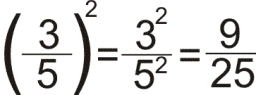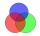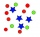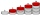# Divide 8

Divide 6840 by x y and z, in such a way that x has twice as much as y, who has half as much as z

Correct result:

x =  2736
y =  1368
z =  2736

#### Solution:

$y=x\mathrm{/}2=2736\mathrm{/}2=1368$We would be pleased if you find an error in the word problem, spelling mistakes, or inaccuracies and send it to us. Thank you!Tips to related online calculators
Do you have a linear equation or system of equations and looking for its solution? Or do you have quadratic equation?

## Next similar math problems:

• Three groupsIn the company, employees are divided into three groups. In the first group, which includes 12% of the company's total number of employees, the average salary is CZK 40,000, in the second group CZK 35,000, in the third group CZK 25,000. The average salary78 school students graduate from mathematics or physics. There are three times more students who graduate from mathematics and do not graduate from physics than those who graduate from physics and do not graduate from mathematics. 69 students graduate fro
• Proportional relationshipThe ordered pairs (6,24) and (1, s) represent a proportional relationship. Find the value of s.
• AndrewAndrew had an offer for a job starting at 100000 per year and guaranteeing her to raise of 10000 a year for the next years. Find her salary for the 4th year.
• StaircaseOn a staircase 3.6 meters high, the number of steps would increase by 3 if the height of one step decreased by 4 cm. How high are the stairs?
• Trapezoid 25Trapezoid PART with AR||PT has (angle P=x) and (angle A=2x) . In addition, PA = AR = RT = s. Find the length of the median of Trapezoid PART in terms of s.
• Up and down motionWe throw the body from a height h = 5 m above the Earth vertically upwards v0 = 10 m/s. How long before we have to let the second body fall freely from the same height to hit the Earth at the same time?
• Perpendicular projectionDetermine the distance of a point B[1, -3] from the perpendicular projection of a point A[3, -2] on a straight line 2 x + y + 1 = 0.
• Sum 1-6Find the sum of the geometric progression 3, 15, 75,… to six terms.
• What is 10What is the 5th term, if the 8th term is 80 and common ratio r =1/2?
• Terms of GPWhat is the 6th term of the GP 9, 81, 729,. .. ?
• Find the 19Find the 1st term of the GP ___, -6, 18, -54.
• Find next memberFind x if the numbers from a GP 7, 49, x.
• Missing term 2What is the missing term for the Geometric Progression (GP) 3, 15, 75,__, 1875?
• If the 3If the 6th term of a GP is 4 and the 10th is 4/81, find common ratio r.
• Sphere cutA sphere segment is cut off from a sphere k with radius r = 1. The volume of the sphere inscribed in this segment is equal to 1/6 of the volume of the segment. What is the distance of the cutting plane from the center of the sphere?
• Insert 4Insert 3 arithmetic means between 3 and 63.